#Quality Digest

Featured Product
This Week in Quality Digest Live
Six Sigma Features
Richard Harpster
Good news? You are probably already doing it.
Donald J. Wheeler
Does your approach do what you need?
James J. Kline
Quality professional organizations need to adjust their body of knowledge to include an understanding of big data
Donald J. Wheeler
In spite of what everyone says to the contrary
Brittney McIver
Every CAPA should begin with investigation
Six Sigma News
Elsmar Cove is a leading forum for quality and standards compliance
Is the future of quality management actually business management?
Too often process enhancements occur in silos where there is little positive impact on the big picture
Collect measurements, visual defect information, simple Go/No-Go situations from any online device
Good quality is adding an average of 11 percent to organizations’ revenue growth
Floor symbols and decals create a SMART floor environment, adding visual organization to any environment
A guide for practitioners and managers
Making lean Six Sigma easier and adaptable to current workplaces
Gain visibility into real-time quality data to improve manufacturing process efficiency, quality, and profitsBio

Six Sigma

## What Is Chunky Data?

### What happens when the measurement increment gets too large?

Published: Tuesday, December 6, 2011 - 15:58

Many times measurements are made using measurement increments which are too large for the job. Fortunately this problem is easily detected by ordinary, production-line process behavior charts. No special studies are necessary; no standard parts or batches are needed. You simply need to recognize the telltale signs. It is the purpose of this column to explain these signs of chunky data, to outline the nature of the problem that causes chunky data, and to suggest what can be done about it when it occurs.

Most problems with process behavior charts are fail-safe. That is, the charts will err in the direction of hiding a signal rather than causing a false alarm. Because of this feature, when you get a signal, you can trust the chart to be guiding you in the right direction. Chunky data is the only exception to this fail-safe feature of the process behavior chart.

Data are said to be chunky when the distance between the possible values becomes too large. For example, what would happen if measurements of the heights of different individuals were made to the nearest yard? Clearly, the variation from person to person would be lost in the round off, and any attempt to characterize the variation in heights would be flawed. When the round off of the measurements begins to obliterate the variation within the data you will have chunky data. The effect that chunky data has upon process behavior charts is illustrated by the following example.

The data in figure 1 are the measurements of a physical dimension on a plastic knob. These data are recorded to the nearest one-thousandth of an inch (0.001 in.). Subgroups 1 to 14 are in the first column, while subgroups 15 to 27 are in the second column. There are no signals of exceptional variation on either the average chart or the range chart. These data show no evidence of a lack of homogeneity, and therefore we would conclude that the process producing these rheostat knobs is being operated predictably.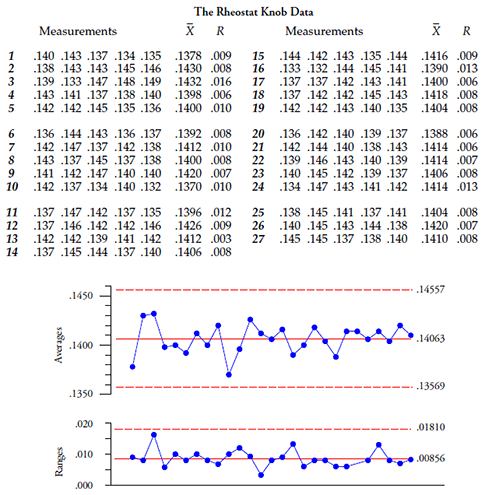Figure 1: Average and range chart when rheostat knob data are recorded to 0.001 in.

The data in figure 2 are the same data as those in figure 1, except that in figure 2 each value has been rounded off to the nearest one-hundredth of an inch (0.01 in.). (This was done merely to illustrate the effect of having a measurement increment that is too large. It is not something that you would do in practice.) After rounding these data, the averages and ranges were recomputed and a new average and range chart was obtained. There we find four averages and two ranges outside the limits in figure 2. The usual interpretation of the chart in figure 2 would be that these data show a lack of homogeneity, and that the underlying process is changing in some manner.

However, we know that the charts in figure 1 and those in figure 2 both represent the same process. The only difference between the two charts is the measurement increment used. Based on figure 1, we have to conclude that the "signals" in figure 2 are actually false alarms created by the round-off operation.Figure 2: Average and range chart when rheostat knob data are rounded to 0.01 in.

Comparing figures 1 and 2 it should be apparent that chunky data can make a predictable process appear to be unpredictable.

Fortunately, it is easy to tell when the data have become chunky. The key is in understanding the differences between figure 1 and figure 2. When we look at the running records for the averages we see that they both vary within the same range from a low near 0.136 to a high near 0.144. When we look at the running records for the ranges we see that they also occupy roughly the same space on the vertical scale, going from 0.000 to 0.020. However, due to the difference in measurement increments, the running records in figure 2 take on fewer values than the running records in figure 1, and the highs and lows in figure 2 are more extreme.

While the running records are trying to tell us the same story in both figure 1 and figure 2, the sparsity of the possible values in figure 2 makes the running records look more "chunky" than those in figure 1. At the same time, the many zero ranges in figure 2 deflate the average range, which in turn deflates the limits.

So while the highs and lows get emphasized by the larger measurement increments, the limits get squeezed. When this happens it is inevitable that the running record and limits will collide and produce an excess number of false alarms.

So how can we spot this problem? The very look of the running records is one clue. The abundance of zero ranges is another. However, the clear-cut, unequivocal indicator of chunky data is the number of possible values for the ranges within the limits on the range chart. Because the ranges will always have the same increments as the original data, the ranges in figure 1 are all multiples of one-thousandth of an inch. The range chart from figure 1 is reproduced in figure 3 with tick marks added to the vertical scale to represent the possible values for the ranges. Inspection of figure 3 will show that there are 19 possible values below the upper range limit. (Your software will not supply these tick marks. You will have to do this mental computation yourself.)Figure 3: Range chart from figure 1

The range chart from figure 2 is given in figure 4. There the ranges are all multiples of one hundredth of an inch, resulting in only two possible values below the upper range limit.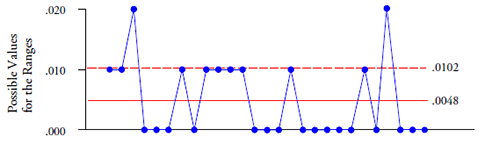Figure 4: Range chart from figure 2

### Chunky data detection rules

Your data can be said to be chunky whenever there are four or fewer possible values within the limits of the range chart. To be safe from the effects of chunky data, you need a minimum of five or more possible values within the limits of the range chart.

The only exception occurs when the range chart is based on subgroup size n = 2. In this case, your data can be said to be chunky whenever there are three or fewer possible values within the limits of the range chart. Here the borderline safe condition occurs when you have at least four possible values within the limits.

Figure 1, with 19 possible values, shows no problem due to chunky data. Figure 2, with only two possible values, shows data that are definitely chunky—the measurement increment is too large for the purposes of creating a useful and meaningful process behavior chart. Since chunky data create false alarms, you cannot safely interpret the "signals" of figure 2 as evidence of exceptional process variation. Thus, while chunky data might be used for inspection, they cannot be used to characterize process behavior.

The problem seen in figure 2 is due to the inability of the measurement increments to properly detect and reflect the process variation. When these measurements are rounded to the nearest 0.01 in., most of the information about variation is lost in the round off. As a result the rounded data have many zero ranges even though the original data had no zero ranges. These zero ranges deflate the average range and tighten the computed limits. At the same time, the greater discreteness for both the averages and the ranges will prevent the running records from shrinking with the limits. Eventually it becomes inevitable that some points will fall outside the artificially tightened limits even though the process itself is predictable.

Therefore, the procedure to check for chunky data consists of three steps:
1. Determine the measurement increment used. This is done by inspecting either the ranges or the original data.
2. Determine the upper and lower limits for the range chart. This is done in the usual manner.
3. Determine how many possible values for the range fall within the range limits, and apply the rules given above.

### Fixing chunky data

Since the problem with chunky data comes from the inability to detect variation within the subgroups, the solution consists of increasing the ability of the measurements to detect that variation.

One way to do this is to use smaller measurement increments. If you have been rounding your measurements too aggressively you can solve the problem of chunky data by simply recording an additional digit for each measurement. Even if there is some uncertainty in that extra digit, its inclusion can actually improve the quality of your data. So, regardless of tradition, if your data are chunky because you have been rounding your measurements, you need to stop rounding and start recording an extra digit. If the current measurement system will not provide you with an additional digit for your observations, then you may need to consider changing the measurement system.

Another solution to the problem of chunky data is to increase the variation within the subgroups. This will increase the ability of your current measurement system to detect variation within the subgroups. With an average chart this will usually involve a change in what a subgroup represents, rather than merely increasing the subgroup size. When a single subgroup represents several successive parts coming off a line, you can usually increase the variation sufficiently by simply expanding the subgroup to represent a longer period of time. When the within-subgroup variation becomes detectable, the visible effects of chunky data on the average and range chart will disappear.

With an XmR chart this approach of increasing the variation is often equivalent to increasing the time period between observations. When this is not feasible, the only alternative is to use smaller measurement increments. An example of the relationship between sample frequency and chunky data is provided by the following.

An automated system could be set to sample the on-line temperature readings at different frequencies. An engineer wanted to have the system produce XmR charts. In order to determine the appropriate sampling frequency, he experimented with several different frequencies. First the temperatures were sampled 28 times per hour (once every 128 seconds) and the values were placed on an XmR chart. The limits for this chart are shown as the first set of yellow bands on the left side of figure 5. (In order to simplify the picture, only the limits will be shown in figure 5.) Because the temperatures were recorded to the nearest degree Celsius, the horizontal lines in figure 5 define the possible values for both the X values and the mR values. With 28 readings per hour, the X chart defined values of 16° to 22° as routine and the moving range (mR) chart had five possible values for the ranges within the limits, hence no problem with chunky data.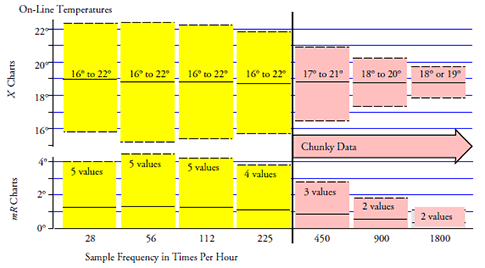Figure 5: Limits for XmR charts for on-line temps at seven frequencies

Next the temperatures were sampled 56 times per hour (once every 64 seconds). This X chart also defined values of 16° to 22° as routine and the mR chart had five possible values for the ranges within the limits. Still no problem with chunky data.

Next the temperatures were sampled 112 times per hour (once every 32 seconds). This X chart also defined values of 16° to 22° as routine and the mR chart had five possible values for the ranges within the limits—still no problem. As long as the variation from one point to another is large enough to allow the moving ranges to reliably characterize the routine process variation, the charts will continue to deliver the same message.

Next the temperatures were sampled 225 times per hour (once every 16 seconds). This X chart has limits that round off to 16° and 22° while the mR chart has only four possible values for the ranges within the limits—a borderline condition. While the limits for the X chart are slightly tighter here than in the previous charts, the message of the XmR chart remains essentially the same as with the previous charts with lower sample frequencies.

With the sample frequency at 450 times per hour (once every 8 seconds) the XmR chart shows only three possible values within the limits on the range chart. This is indicative of chunky data, and we can see how the limits begin to shrink.

At 900 samples per hour the problem just gets worse—the limits are tightened even more.

At 1800 samples per hour the limits threaten to disappear.

So while the temperatures may be sampled very rapidly, meaningful limits cannot be constructed from these data when the sample frequency exceeds 225 times per hour.

When the borderline condition is passed, the limits begin to shrink in response to the increased number of zero ranges, and the limits will always be artificially tight. Whenever the chart displays chunky data, the tightened limits will result in an increased number of false alarms, which will undermine any attempt to interpret points outside the limits.

Increasing the subgroup size may reduce the number of false alarms on the average chart, but it will not reliably remedy the basic problem of chunky data, which comes from the round off deflating the estimate of dispersion.

If your observations consist of counts, and those counts display the effects of chunky data when placed on an XmR chart, then your data are irredeemably chunky (and you are probably counting rare events). Such data may be used to create running records, but they will not support the computation of meaningful limits.

### The basis for the detection rules

The problem of chunky data is the problem of estimating the variation within the data when the measurement increment gets too large. To understand this problem and to show the basis for the rules for detecting chunky data, we will return to the data of figures 1 and 2.

When an average range statistic is divided by the appropriate bias correction factor, d2, we will obtain an unbiased estimate of the within-subgroup dispersion: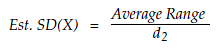To illustrate how this works consider using the first nine subgroups of figure 1 to obtain an average range of 0.009111. Then, using the next nine subgroups we obtain a second average range of 0.008667. Finally, using the last nine subgroups we find a third average range of 0.007889. For subgroups of size five, the bias correction factor is 2.326. Thus, our three average ranges can be used to obtain three estimates of SD(X). These are, respectively, 0.00392, 0.00373, and 0.00339. These three values are shown along with their theoretical distribution in figure 6. In creating figure 6, I used a value for SD(X) of 0.00368. Therefore, in this case the measurement increment of 0.001 can be said to be 0.272 SD(X).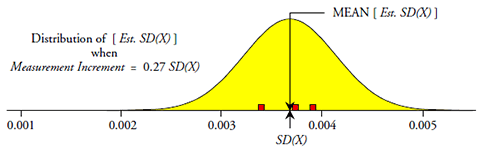Figure 6: The distribution of unbiased range-based estimates of SD(X) from figure 1

If we continued to collect data from the predictable process of figure 1 and used these data to compute estimates of SD(X) (using average ranges based on nine subgroups of size five), then we would eventually end up with a histogram that approximates the distribution shown in figure 6. This theoretical distribution has a mean value that is equal to SD(X).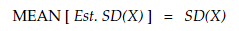On the other hand, in figure 2 the measurement increment is 0.01, which is 2.72 SD(X), and the estimates of SD(X) are no longer centered on SD(X). To illustrate this we divide the data of figure 2 into three sets consisting of nine subgroups of size five and obtain average ranges of 0.00667, 0.00444, and 0.00333 respectively. These values yield estimates of SD(X) of 0.00287, 0.00191, and 0.00143. These three values are shown along with their theoretical distribution in figure 7. Here the mean value of the distribution is no longer equal to the value of SD(X).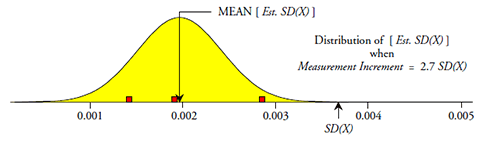Figure 7: The distribution of biased range-based estimates of SD(X) from figure 2

In figure 6 the ratio of MEAN[Est. SD(X)] to SD(X) is 1.000. In figure 7 this ratio is much less than 1.000. Figure 8 uses the ratio of MEAN[Est. SD(X)] to SD(X) to show how large measurement increments introduce bias into the estimates of dispersion. The blue triangle on the left represents the situation in figure 6, while the blue triangle on the right represents the situation in figure 7. The bottom curve is for the average of two-point moving ranges. The remaining curves, in ascending order according to the right-hand end points, are for average ranges based on subgroups of size 2, 3, 4, 5, 6, 8, and 10.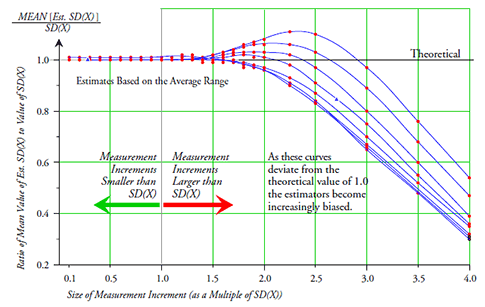Figure 8: How the measurement increment affects range-based estimates of SD(X)

On the left-hand side of figure 8 all of the curves are near 1.00. This is what theory predicts should happen. Here the measurement increments do not interfere with the computations and the theoretical relationships still work in practice. However, as the measurement increment gets larger than SD(X), these curves begin to move around with substantial departures occurring when the measurement increment exceeds 1.5 SD(X). These departures from the theoretical mean that the unbiased estimators of dispersion will become biased. As a result, the formulas and computations based upon the theoretical relationships will be undermined.

Thus, when the measurement increment is less than or equal to SD(X), the theoretical relationships will hold and the usual formulas built on those relationships will work as advertised. However, as the measurement increment gets larger than SD(X), there will come a point where the usual formulas will no longer work.

If we define the borderline safe condition to be that point at which the measurement increment is equal to the value of SD(X), then the limits for the range chart will have the following form: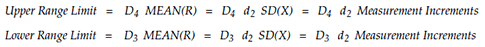These values are tabled for subgroup sizes of n = 2 to n = 10 in figure 9. Consideration of these limits reveals the number of possible values within the limits on a range chart at this borderline safe condition.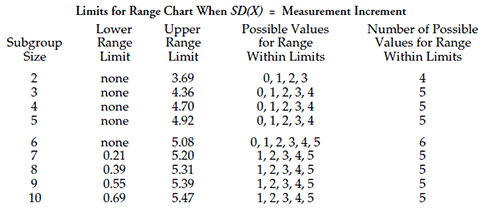Figure 9: Table for the number of possible values within the limits on a range chart

Since the values in figure 9 define the borderline safe condition, the following guidelines for detecting chunky data are established:

The measurement increment borders on being too large when there are only five possible values within the limits on the range chart. Four values within the limits will be indicative of chunky data, and fewer than four values will severely distort the limits. The only notable exception to this occurs when the subgroup size for the range chart is n = 2; here four possible values within the limits on the range chart will represent the borderline safe condition. Three possible values within the limits will be indicative of chunky data, and fewer than three values will result in appreciable distortion of the limits.

While these detection rules will work with range charts and mR charts, they will not work with other charts for dispersion. This is because the range is the only measure of dispersion that preserves the discreteness of the original measurements.

Thus, there need be no confusion about whether or not the measurement increment being used is sufficiently small for the application at hand. The range chart clearly shows when it is not. Fortunately, when this problem exists, the solutions are straightforward: either smaller measurement increments must be used, or the variation within the subgroups must be increased. You must implement one of these solutions before your process behavior charts will be of any real use. If neither of these solutions can be applied, then the data may still be plotted in a running record, and used in a descriptive sense, but they should not be used to compute limits for a process behavior chart.

### Will the standard deviation statistic fix chunky data?

Can we remedy the problem of chunky data by using the standard deviation statistic in place of the range? In addressing this question we need to begin with the observation that for subgroups of size n = 2, the standard deviation statistic is simply the range divided by the square root of 2. Because division by a constant will not change the mathematical properties of a random variable, we will not gain anything by shifting to the standard deviation statistic when n = 2.

For n = 3 or more, when the range chart shows signs of chunkiness, you can make these signs disappear by simply switching to the standard deviation chart. But while the symptoms may vanish, the problem still persists. This may be seen in figure 10 where we see how the measurement increment affects the ability of the average standard deviation statistic to provide a reliable estimate of SD(X).Figure 10: How the measurement increment affects the average standard deviation statistic

As before, with small measurement increments the curves are equal to the theoretical value of 1.00. But as the measurement increment increases in size the curves begin to deviate from 1.00. The bottom curve is for n = 3. The rest of the curves, in ascending order according to their right-hand end points are for n = 4, 5, 6, 8, and 10. Unlike the range-based estimates of SD(X), the standard deviation-based estimates begin to be inflated when the measurement increment gets larger than 0.5 SD(X). However, like the range-based estimates, these estimates all begin to plummet when the measurement increment exceeds twice the value of SD(X). Therefore, the fact that the standard deviation chart does not show the effects of chunky data does not mean that those effects have been eliminated. It simply means that the complex structure of the standard deviation statistic has hidden the chunkiness.

Figures 8 and 10 show that the effects of chunky data are eventually the same regardless of whether we are using range-based estimates or standard deviation-based estimates. Once the measurement increment exceeds 2.0 SD(X) all estimates will plummet toward zero.

Thus the problem of chunky data is a problem that you will need to recognize to avoid having an excess number of false alarms. In this regard the range chart makes it possible to spot and test for chunky data while the standard deviation chart does not. Moreover, the average range does a better job of providing an unbiased estimator on the edges of chunky data than does the average standard deviation statistic. So not only are there no advantages to using the standard deviation statistic, but there are some practical disadvantages as well.

### Summary

Chunky data is one problem with the measurement system that can be detected on an ordinary process behavior chart. It is easy to spot, and it is important to know about because it represents the one failure mode for a process behavior chart where the chart does not fail safely. Chunky data will eventually create an excess number of false alarms, which will undermine the credibility of process behavior chart.

Chunky data will undermine our ability to use the data to compute appropriate limits for a process behavior chart. Chunky data may still be plotted on a running record, and they may still be used for inspection, but they are inadequate for use on a process behavior chart.

Theoretical relationships always assume that the measurements are continuous, and all formulas for computations are based on these theoretical relationships. In practice our measurements are never continuous. When the measurement increment gets large enough it will contaminate the computations and result in formulas that do not work as advertised. Fortunately, if you begin your data analysis as you should, and use a process behavior chart as the first step in that analysis, you can check for this problem before it gives you flawed results.

### Discuss### Donald J. Wheeler

Find out about Dr. Wheeler’s virtual seminars for 2022 at www.spcpress.com. Dr. Wheeler is a fellow of both the American Statistical Association and the American Society for Quality who has taught more than 1,000 seminars in 17 countries on six continents. He welcomes your questions; you can contact him at djwheeler@spcpress.com.